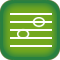Intervals I: Perfect Intervals

Skills covered:
 UnisonsOctaves Perfect fifthsPerfect fourths

1.  Introduction to Intervals
Lesson

2.  Unisons and octaves
Lesson

3.  Perfect fifths
Lesson

4.  Aural recognition of P1, P5 and P8 (melodic)
Lesson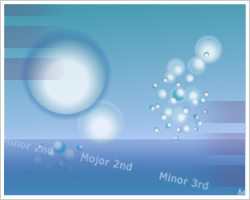Melodic Drops
Level 1

5.  Aural recognition of P1, P5 and P8 (harmonic)
Lesson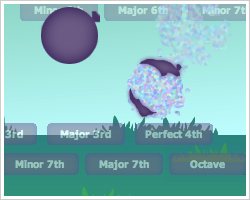Harmonic Drops
Level 1

6.  P1, P5 and P8 in major keys (solfege)
LessonTwo Tones (Major)
Level 1

7.  Perfect fourths
Lesson

8.  Aural recognition of P1, P4 and P8 (melodic)
LessonMelodic Drops
Level 2

9.  Aural recognition of P1, P4 and P8 (harmonic)
LessonHarmonic Drops
Level 2

10.  P1, P4 and P8 in major keys (solfege)
LessonTwo Tones (Major)
Level 2

11.  Aural recognition of perfect intervals (melodic) 1
LessonMelodic Drops
Level 3

12.  Aural recognition of perfect intervals (harmonic) 1
LessonHarmonic Drops
Level 3

13.  Notation of perfect intervals
Lesson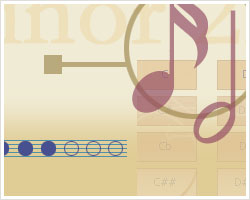Flash Notation (Intervals)
Level 1

14.  P1, P4, P5 and P8 in major keys (solfege)
LessonTwo Tones (Major)
Level 3

15.  Aural recognition of perfect intervals (melodic) 2
Lesson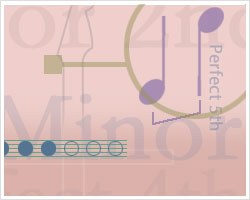Flash Intervals (Mel.)
Level 1

16.  Aural recognition of perfect intervals (harmonic) 2
LessonFlash Intervals (Harmonic)
Level 1